## 归并排序(Merge Sort)的原理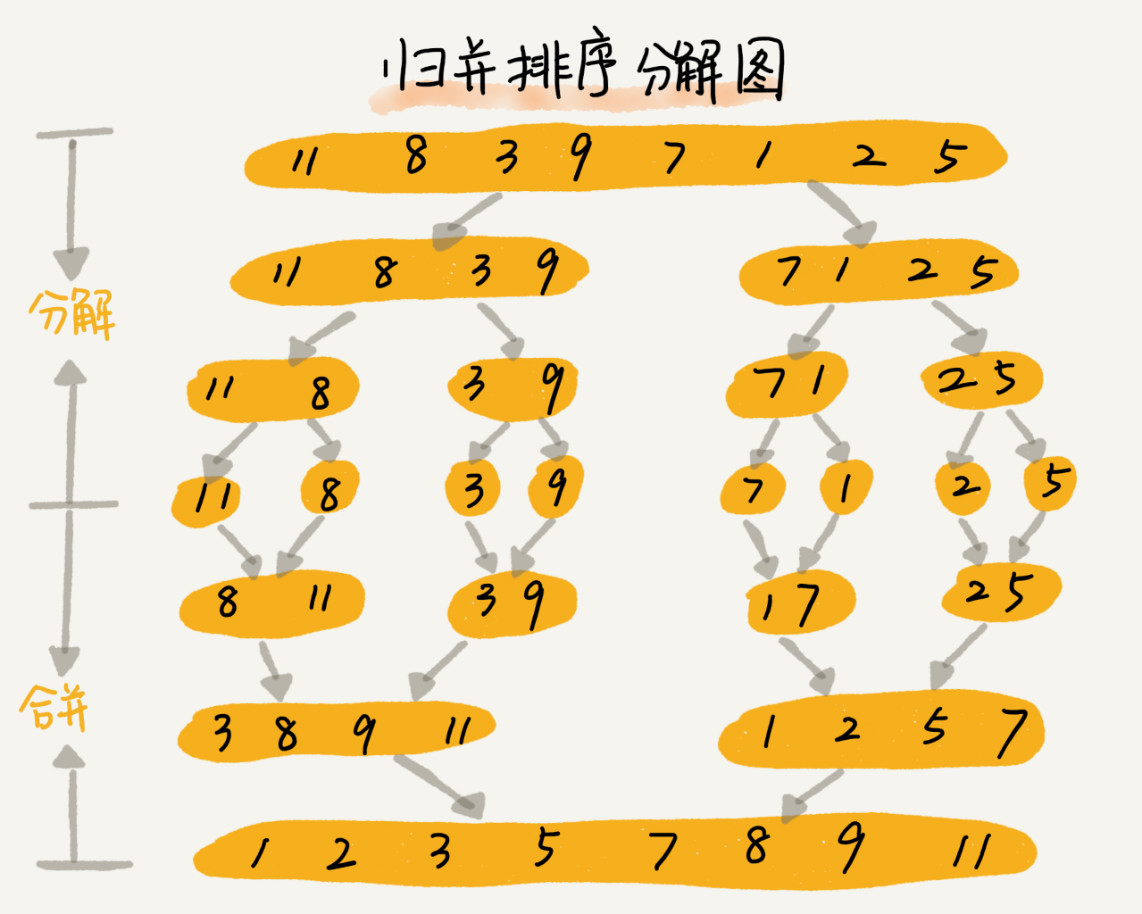递推公式：
merge_sort(p…r) = merge(merge_sort(p…q), merge_sort(q+1…r))

p >= r 不用再继续分解

merge_sort(p…r) 表示，给下标从 pr 之间的数组排序。将这个排序问题转化为了两个子问题，merge_sort(p…q)merge_sort(q+1…r)，其中下标 q 等于 pr 的中间位置，也就是 (p+r)/2。当下标从 pq 和从 q+1r 这两个子数组都排好序之后，再将两个有序的子数组合并在一起，这样下标从 pr 之间的数据就也排好序了。

// 归并排序算法, A 是数组，n 表示数组大小
merge_sort(A, n) {
merge_sort_c(A, 0, n-1)
}

// 递归调用函数
merge_sort_c(A, p, r) {
// 递归终止条件
if p >= r  then return

// 取 p 到 r 之间的中间位置 q
q = (p+r) / 2
// 分治递归
merge_sort_c(A, p, q)
merge_sort_c(A, q+1, r)
// 将 A[p...q] 和 A[q+1...r] 合并为 A[p...r]
merge(A[p...r], A[p...q], A[q+1...r])
}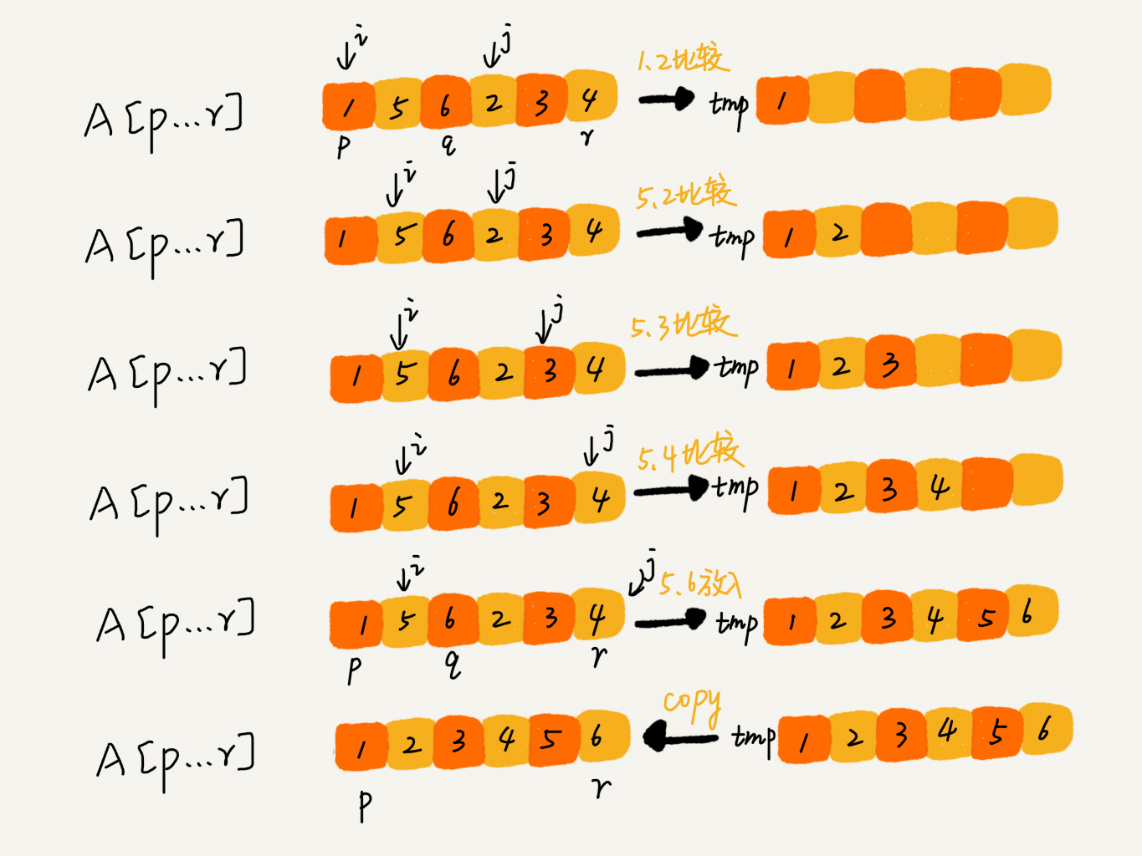merge() 函数写成伪代码，就是下面这样：

merge(A[p...r], A[p...q], A[q+1...r]) {
var i := p，j := q+1，k := 0 // 初始化变量 i, j, k
var tmp := new array[0...r-p] // 申请一个大小跟 A[p...r] 一样的临时数组
while i<=q AND j<=r do {
if A[i] <= A[j] {
tmp[k++] = A[i++] // i++ 等于 i:=i+1
} else {
tmp[k++] = A[j++]
}
}

// 判断哪个子数组中有剩余的数据
var start := i，end := q
if j<=r then start := j, end:=r

// 将剩余的数据拷贝到临时数组 tmp
while start <= end do {
tmp[k++] = A[start++]
}

// 将 tmp 中的数组拷贝回 A[p...r]
for i:=0 to r-p do {
A[p+i] = tmp[i]
}
}

## 归并排序的性能分析

T(a) = T(b) + T(c) + K

T(1) = C；   n=1 时，只需要常量级的执行时间，所以表示为 C。
T(n) = 2*T(n/2) + n； n>1

T(n) = 2*T(n/2) + n
= 2*(2*T(n/4) + n/2) + n = 4*T(n/4) + 2*n
= 4*(2*T(n/8) + n/4) + 2*n = 8*T(n/8) + 3*n
= 8*(2*T(n/16) + n/8) + 3*n = 16*T(n/16) + 4*n
......
= 2^k * T(n/2^k) + k * n
......

## 快速排序（Quicksort）的原理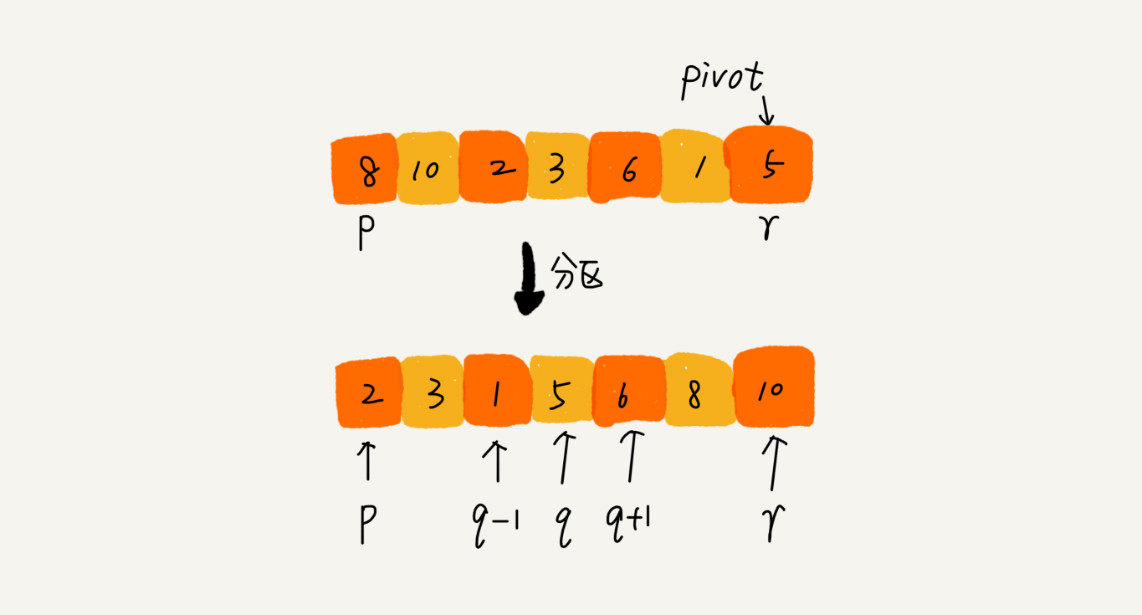递推公式：
quick_sort(p…r) = quick_sort(p…q-1) + quick_sort(q+1, r)

p >= r

// 快速排序，A 是数组，n 表示数组的大小
quick_sort(A, n) {
quick_sort_c(A, 0, n-1)
}
// 快速排序递归函数，p,r 为下标
quick_sort_c(A, p, r) {
if p >= r then return

q = partition(A, p, r) // 获取分区点
quick_sort_c(A, p, q-1)
quick_sort_c(A, q+1, r)
}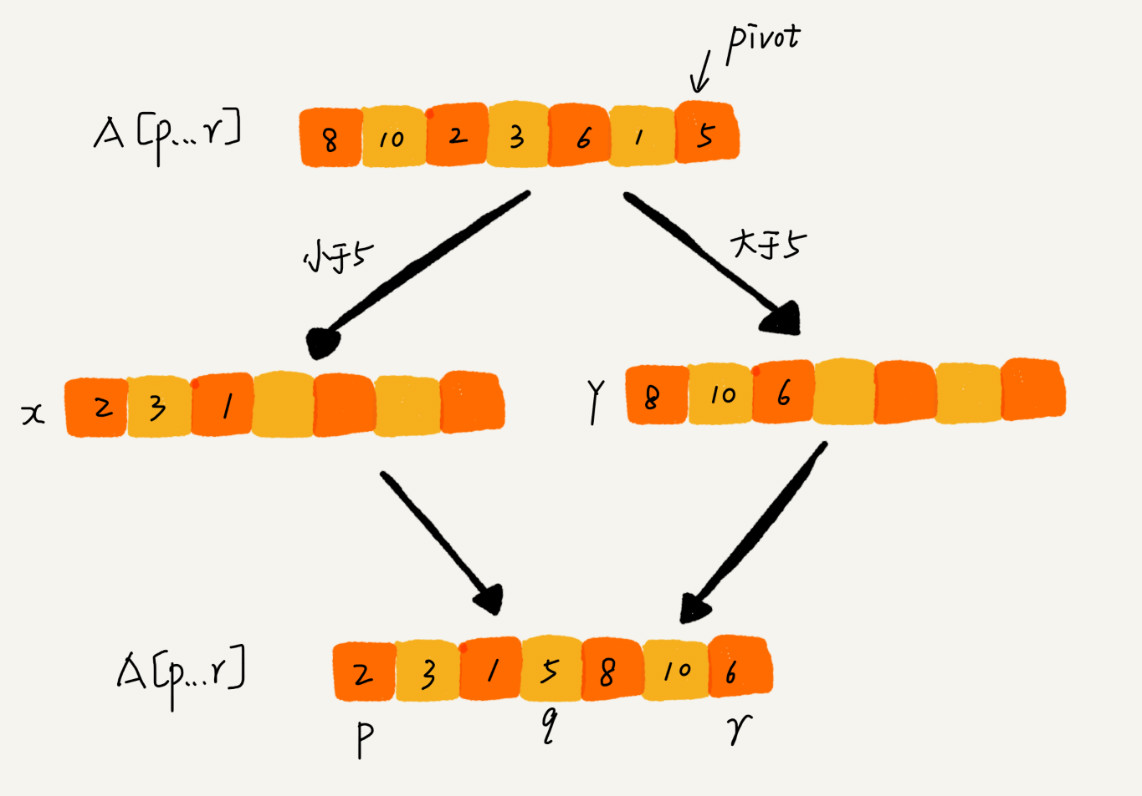partition(A, p, r) {
pivot := A[r]
i := p
for j := p to r-1 do {
if A[j] < pivot {
swap A[i] with A[j]
i := i+1
}
}
swap A[i] with A[r]
return i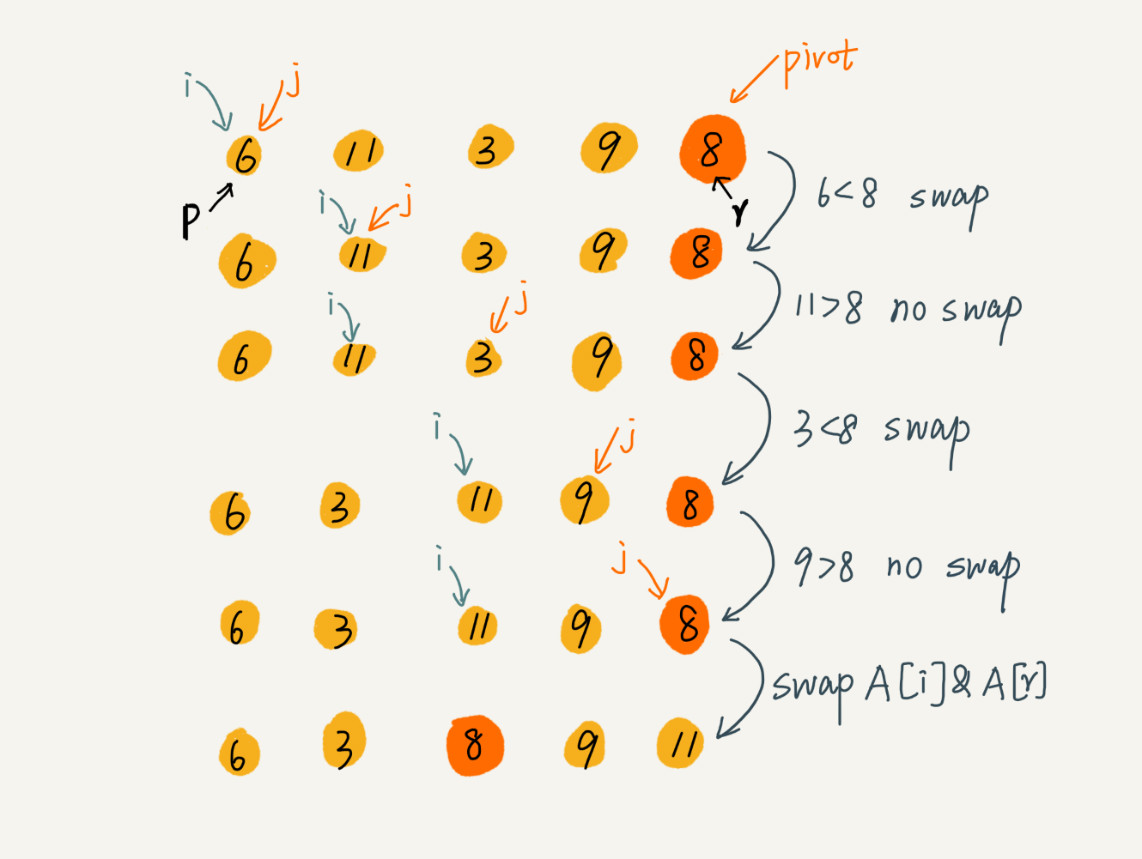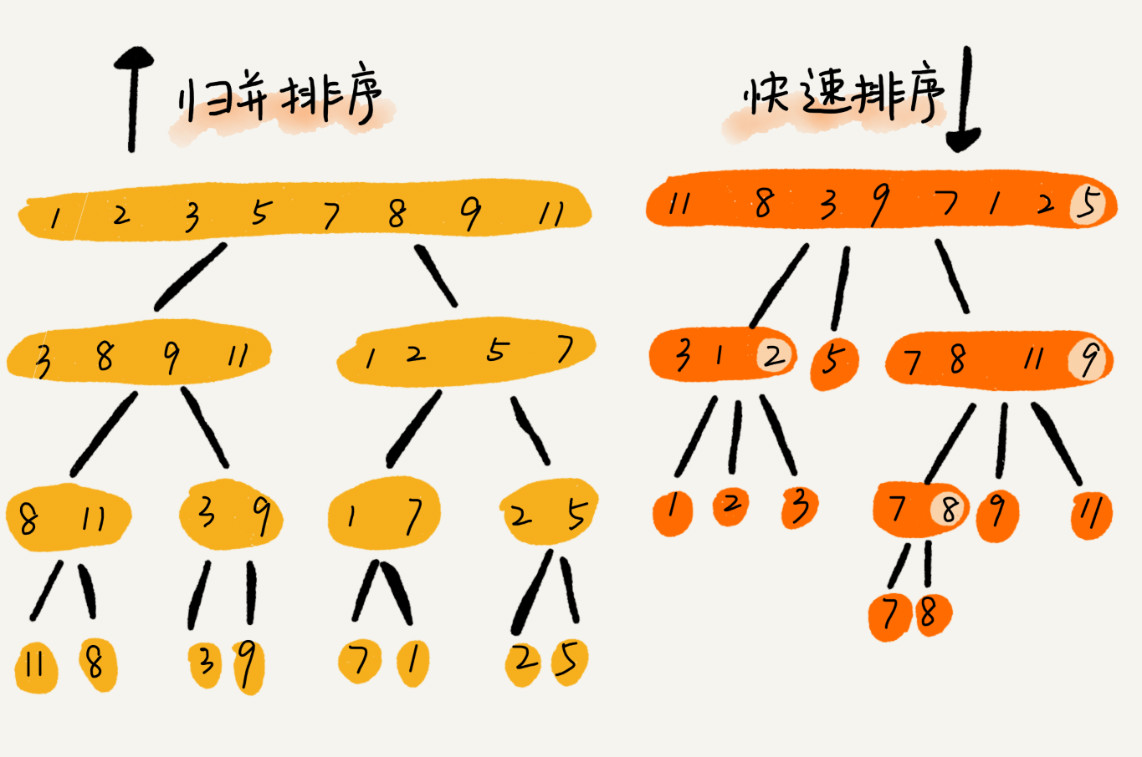## 快速排序的性能分析

T(1) = C；   n=1 时，只需要常量级的执行时间，所以表示为 C。
T(n) = 2*T(n/2) + n； n>1

T(1) = C；   n=1 时，只需要常量级的执行时间，所以表示为 C。

T(n) = T(n/10) + T(9*n/10) + n； n>1

## 思考

• **$O(n)$时间复杂度内求无序数组中第K大元素?**比如4，2，5，12，3这样一组数据，第3大元素是4

选择数组区间 A[0…n-1] 的最后一个元素 A[n-1] 作为 pivot，对数组 A[0…n-1] 原地分区，这样数组就分成了三部分，A[0…p-1]A[p]A[p+1…n-1]

如果 p+1=K，那 A[p] 就是要求解的元素；如果 K>p+1, 说明第 K 大元素出现在 A[p+1…n-1] 区间，再按照上面的思路递归地在 A[p+1…n-1] 这个区间内查找。同理，如果 K<p+1，那就在 A[0…p-1] 区间查找。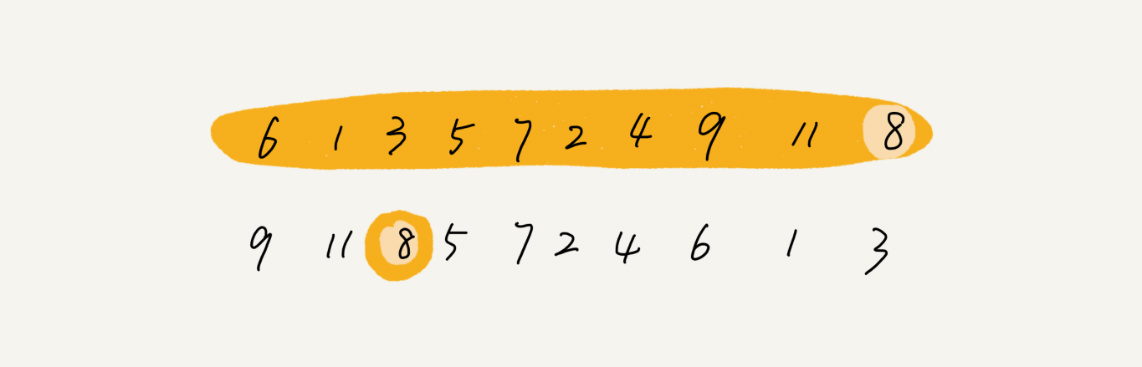再来看，为什么上述解决思路的时间复杂度是 $O(n)$？

第一次分区查找，需要对大小为 n 的数组执行分区操作，需要遍历 n 个元素。第二次分区查找，只需要对大小为 n/2 的数组执行分区操作，需要遍历 n/2 个元素。依次类推，分区遍历元素的个数分别为、n/2n/4n/8n/16.……直到区间缩小为 1

如果把每次分区遍历的元素个数加起来，就是：n+n/2+n/4+n/8+…+1。这是一个等比数列求和，最后的和等于 2n-1。所以，上述解决思路的时间复杂度就为 $O(n)$。

你可能会说，有个很笨的办法，每次取数组中的最小值，将其移动到数组的最前面，然后在剩下的数组中继续找最小值，以此类推，执行 K 次，找到的数据不就是第 K 大元素了吗？

不过，时间复杂度就并不是 $O(n)$ 了，而是 $O(K * n)$。你可能会说，时间复杂度前面的系数不是可以忽略吗？$O(K * n)$ 不就等于 $O(n)$ 吗？

这个可不能这么简单地划等号。当 K 是比较小的常量时，比如 12，那最好时间复杂度确实是 $O(n)$；但当 K 等于 n/2 或者 n 时，这种最坏情况下的时间复杂度就是 $O(n^2)$ 了。

• 10个访问日志文件，每个日志文件大小约为300MB，每个文件里的日志都是按照时间戳从小到大排序的。现在需要将这10个较小的日志文件合并为1个日志文件，合并之后的日志仍然按照时间戳从小到大排列。如果处理上述任务的机器内存只有1GB，你有什么好的解决思路能快速地将这10个日志文件合并？

每次从各个文件中取一条数据，在内存中根据数据时间戳构建一个最小堆，然后每次把最小值给写入新文件，同时将最小值来自的那个文件再出来一个数据，加入到最小堆中。
这个空间复杂度为常数，但没能很好利用1G内存，而且磁盘单个读取比较慢，所以考虑每次读取一批数据，没了再从磁盘中取，时间复杂度还是一样$O(n)$。

## 归并排序 Java 实现代码：

public class MergeSort {

// 归并排序算法, a是数组，n表示数组大小
public static void mergeSort(int[] a, int n) {
mergeSortInternally(a, 0, n-1);
}

// 递归调用函数
private static void mergeSortInternally(int[] a, int p, int r) {
// 递归终止条件
if (p >= r) return;

// 取p到r之间的中间位置q,防止（p+r）的和超过int类型最大值
int q = p + (r - p)/2;
// 分治递归
mergeSortInternally(a, p, q);
mergeSortInternally(a, q+1, r);

// 将A[p...q]和A[q+1...r]合并为A[p...r]
merge(a, p, q, r);
}

private static void merge(int[] a, int p, int q, int r) {
int i = p;
int j = q+1;
int k = 0; // 初始化变量i, j, k
int[] tmp = new int[r-p+1]; // 申请一个大小跟a[p...r]一样的临时数组
while (i<=q && j<=r) {
if (a[i] <= a[j]) {
tmp[k++] = a[i++]; // i++等于i:=i+1
} else {
tmp[k++] = a[j++];
}
}

// 判断哪个子数组中有剩余的数据
int start = i;
int end = q;
if (j <= r) {
start = j;
end = r;
}

// 将剩余的数据拷贝到临时数组tmp
while (start <= end) {
tmp[k++] = a[start++];
}

// 将tmp中的数组拷贝回a[p...r]
for (i = 0; i <= r-p; ++i) {
a[p+i] = tmp[i];
}
}

/**
* 合并(哨兵)
*
* @param arr
* @param p
* @param q
* @param r
*/
private static void mergeBySentry(int[] arr, int p, int q, int r) {
int[] leftArr = new int[q - p + 2];
int[] rightArr = new int[r - q + 1];

for (int i = 0; i <= q - p; i++) {
leftArr[i] = arr[p + i];
}
// 第一个数组添加哨兵（最大值）
leftArr[q - p + 1] = Integer.MAX_VALUE;

for (int i = 0; i < r - q; i++) {
rightArr[i] = arr[q + 1 + i];
}
// 第二个数组添加哨兵（最大值）
rightArr[r-q] = Integer.MAX_VALUE;

int i = 0;
int j = 0;
int k = p;
while (k <= r) {
// 当左边数组到达哨兵值时，i不再增加，直到右边数组读取完剩余值，同理右边数组也一样
if (leftArr[i] <= rightArr[j]) {
arr[k++] = leftArr[i++];
} else {
arr[k++] = rightArr[j++];
}
}
}
}

## 快速排序 Java 实现代码：

public class QuickSort {

// 快速排序，a是数组，n表示数组的大小
public static void quickSort(int[] a, int n) {
quickSortInternally(a, 0, n-1);
}

// 快速排序递归函数，p,r为下标
private static void quickSortInternally(int[] a, int p, int r) {
if (p >= r) return;

int q = partition(a, p, r); // 获取分区点
quickSortInternally(a, p, q-1);
quickSortInternally(a, q+1, r);
}

private static int partition(int[] a, int p, int r) {
int pivot = a[r];
int i = p;
for(int j = p; j < r; ++j) {
if (a[j] < pivot) {
if (i == j) {
++i;
} else {
int tmp = a[i];
a[i++] = a[j];
a[j] = tmp;
}
}
}

int tmp = a[i];
a[i] = a[r];
a[r] = tmp;

System.out.println("i=" + i);
return i;
}
}